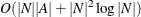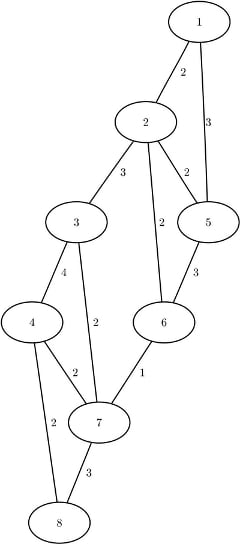### Minimum Cut

A cut is a partition of the nodes of a graph into two disjoint subsets. The cut-set is the set of links whose from and to nodes are in different subsets of the partition. A minimum cut of an undirected graph is a cut whose cut-set has the smallest link metric, which is measured as follows: For an unweighted graph, the link metric is the number of links in the cut-set. For a weighted graph, the link metric is the sum of the link weights in the cut-set.

In PROC OPTNET, the minimum cut algorithm can be invoked by using the experimental MINCUT statement. The options for this statement are described in the section MINCUT Statement. This algorithm can be used only on undirected graphs.

If the value of the MAXNUMCUTS= option is greater than 1, then the algorithm can return more than one set of cuts. The resulting cuts can be described in terms of partitions of the nodes of the graph or the links in the cut-sets. The node partition is specified by the ```mincut_``` variable, for each cut, in the data set that is specified in the OUT_NODES= option in the PROC OPTNET statement. Each node is assigned the value 0 or 1, which defines the side of the partition to which it belongs. The cut-set is defined in the output data set that is specified in the OUT= option in the MINCUT statement. This data set lists the links and their weights for each cut.

PROC OPTNET uses the Stoer-Wagner algorithm (Stoer and Wagner 1997) to compute the minimum cuts. This algorithm runs in time.

#### Minimum Cut for a Simple Undirected Graph

As a simple example, consider the weighted undirected graph in Figure 2.35.

Figure 2.35: A Simple Undirected GraphThe links data set can be represented as follows:

```data LinkSetIn;
input from to weight @@;
datalines;
1 2 2  1 5 3  2 3 3  2 5 2  2 6 2
3 4 4  3 7 2  4 7 2  4 8 2  5 6 3
6 7 1  7 8 3
;
```

The following statements calculate minimum cuts in the graph and output the results in the data set `MinCut`:

```proc optnet
loglevel     = moderate
out_nodes    = NodeSetOut
mincut
out        = MinCut
maxnumcuts = 3;
run;
%put &_OROPTNET_;
%put &_OROPTNET_MINCUT_;
```

The progress of the procedure is shown in Figure 2.36.

Figure 2.36: PROC OPTNET Log for Minimum Cut

 NOTE: ------------------------------------------------------------------------- NOTE: ------------------------------------------------------------------------- NOTE: Running OPTNET. NOTE: ------------------------------------------------------------------------- NOTE: ------------------------------------------------------------------------- NOTE: Reading the links data set. NOTE: There were 12 observations read from the data set WORK.LINKSETIN. NOTE: Data input used 0.00 (cpu: 0.00) seconds. NOTE: Building the input graph storage used 0.00 (cpu: 0.00) seconds. NOTE: The input graph storage is using 0.0 MBs of memory. NOTE: The number of nodes in the input graph is 8. NOTE: The number of links in the input graph is 12. NOTE: ------------------------------------------------------------------------- NOTE: ------------------------------------------------------------------------- NOTE: Processing MINCUT statement. NOTE: The MINCUT algorithm is experimental in this release. NOTE: The minimum cut algorithm found 3 cuts. NOTE: The cut 1 has weight 4. NOTE: The cut 2 has weight 5. NOTE: The cut 3 has weight 5. NOTE: Processing the minimum cut used 0.00 (cpu: 0.00) seconds. NOTE: ------------------------------------------------------------------------- NOTE: ------------------------------------------------------------------------- NOTE: Creating nodes data set output. NOTE: Creating minimum cut data set output. NOTE: Data output used 0.02 (cpu: 0.02) seconds. NOTE: ------------------------------------------------------------------------- NOTE: ------------------------------------------------------------------------- NOTE: The data set WORK.NODESETOUT has 8 observations and 4 variables. NOTE: The data set WORK.MINCUT has 6 observations and 4 variables. STATUS=OK  MINCUT=OK STATUS=OK  CPU_TIME=0.00  REAL_TIME=0.00

The data set `NodeSetOut` now contains the partition of the nodes for each cut, shown in Figure 2.37.

Figure 2.37: Minimum Cut Node Partition

node mincut_1 mincut_2 mincut_3
1 1 1 1
2 1 1 0
5 1 1 0
3 0 1 0
6 1 1 0
4 0 1 0
7 0 1 0
8 0 0 0

The data set `MinCut` contains the links in the cut-sets for each cut. This data set is shown in Figure 2.38 along with each cut separately.

Figure 2.38: Minimum Cut Sets

mincut from to weight
1 2 3 3
1 6 7 1
2 4 8 2
2 7 8 3
3 1 2 2
3 1 5 3

from to weight
2 3 3
6 7 1
mincut   4

from to weight
4 8 2
7 8 3
mincut   5

from to weight
1 2 2
1 5 3
mincut   5
14